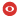Statut Confirmé Série COURS Domaines math.AG Date Vendredi 12 Janvier 2018 Heure 10:00 Institut IPHT Salle Salle Claude Itzykson, Bât. 774 Nom de l'orateur Bertrand Eynard Prenom de l'orateur Addresse email de l'orateur Institution de l'orateur IPhT Titre Riemann surfaces (1/5) Résumé Algebraic equations are widespread in mathematics and physics, and the geometry of their spaces of solutions can be complicated. In the case of an equation of two complex variables, the space of solutions is a Riemann surface. \par We will provide basic tools (going back to Riemann) for studying algebraic equations and describing the geometry of compact Riemann surfaces. \par We will consider a Riemann surface defined from the solution locus of a polynomial equation $P(x,y)=0$ in $\mathbb{C} \times \mathbb{C}$. We will study its topology and geometry, and learn how to integrate differential forms along closed contours. Then we will describe the moduli space of Riemann surfaces with a given topology: its dimension, topology, etc. \par We will introduce some of the many tools that have been invented since the time of Riemann for studying these objects. We will partly follow the Mumford Tata lectures, the Fay lectures, and the Farkas-Kra book. \\ \\ The plan is: \\ - Compact Riemann surfaces, charts, atlas, toplogy. Meromorphic functions and one-forms. Theorems on poles and residues. Newton's polygon. \\ - Integrals, periods, Abel map, Jacobian, divisors. Theta functions, prime form, fundamental form. Basis of cycles, homology and cohomology. \\ - Moduli spaces of Riemann surfaces. Deligne-Mumford compactification, Chern classes, tautological ring. Kontsevich integral and KdV hierarchy. \\ - If times permits: fiber bundles, Hitchin systems, link to integrable systems. Numéro de preprint arXiv Commentaires https://courses.ipht.cnrs.fr/?q=fr/node/195 Fichiers attachés 2017-2018.pdf (4503344 bytes)2018_Eynard_0.pdf (4424243 bytes)Pour obtenir l' affiche de ce séminaire : [ Postscript | PDF ]

 [ Annonces ]    [ Abonnements ]    [ Archive ]    [ Aide ]    [ JavaScript requis ] [ English version ]